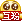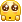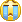直方图下最大矩形面积问题【算法优化向】

2014年3月24日 发表评论 阅读评论好像好久没写算法问题了。。。。上一次写好像是。。额。。大概是去年的事了吧。。。以前还兴致勃勃的狂写Euler Project的个人解答系列，但是后来发现官方有说，请不要在互联网上直接贴关于解答的代码，额，然后他这么一说，这个系列我就不怎么想弄了。。除了一方面不想做这些会被官方讨厌的事情外，其次是。。。懒。。。自己做完题还要花时间上来写思路，各种分析，敲数学公式什么的。。

方法一：暴解def largestRectangleArea(a):
maxarea = 0;
N = len(a);
for i in range(N):
j1 = -1;
for j in range(i-1,-1,-1):
if a[j] < a[i]:
j1 = j;
break;
j2 = N;
for j in range(i+1,N):
if a[j] < a[i]:
j2 = j;
break;
area = a[i]*(j2-j1-1);
if area > maxarea:
maxarea = area;
return maxarea;

方法二：分治

• 包含这个最小的柱子,最大面积是a[i]*n 【下图绿色】
• 出现在最小值左边 【下图左边红色框内某处】
• 出现在最小值右边 【下图右边红色框内某处】def largestRectangleArea(a):
N = len(a);
idx = a.index(min(a));

value1 = a[idx]*N;

if idx != 0:
value2 = largestRectangleArea(a[0:idx]);
else:
value2 = 0;

if idx != N-1:
value3 = largestRectangleArea(a[idx+1:N]);
else:
value3 = 0;

return max([value1,value2,value3]);

方法三：堆栈

1.新建一个空堆栈；栈顶的值标记为$top$.

2.扫描$i$，从$0$$n-1$

• 如果堆栈为空，或者$a[i]$比栈顶$a[top]$的值大，那么把$i$入栈。这个就是上面所说的：如果还没有找到右边第一个比$a[i]$小的值的时候，就先把这个问题保存下来，暂时不算。
• 如果$a[i]$比栈顶$a[top]$的值小，那么说明对于$a[top]$而言，已经找到了右边第一个比它小的值了，就是$a[i]$，什么，你问左边，栈顶往下数的下一个数必然就是左边第一个比它小的值啊！这样就可以算出对于$a[top]$而言，将其作为矩形最小值的话，最大的面积是多少了！这个时候你所需要做的事情就是：
• 计算出这个面积，和当前找到的最大面积对比
• 把栈顶的值弹出
• 如果这个时候$a[top]$的值还比$a[i]$大，继续这么处理，直到$a[top]$$a[i]$小为止。
• $i$入栈

3.扫描完后，一般情况下你会剩下一个单调递增的堆栈，那么一个一个出栈计算面积就可以了。左边$j_1$依旧还是栈顶下面那个值，右边$j_2$这个时候就不存在了，说明每个栈顶的值都是矩形的最右边了。

例子1.堆栈[ ]，开始扫描i从0到7；

2.$i=0$,因为堆栈为空，把0入栈【此时堆栈】

3.$i=1$,因为$a[i]=2 < a[top]=a=5$，所以这个时候栈顶0出栈，并且计算$a$作为矩形最小高度的面积，因为堆栈已空，所以左边界$j_1$就是0，右边界$j_2$就是$i-1=0$；所以最大面积就是$a\times (j_2-j_1+1)=5$;【此时堆栈[ ]】然后再把$i=1$入栈。【此时堆栈】4.$i=2$,因为$a[i]=3>a[top]=a=2$，所以$i=2$入栈。【此时堆栈[1,2]】

5:$i=3$,因为$a[i]=5>a[top]=a=3$，所以$i=3$入栈。【此时堆栈[1,2,3]】

6:$i=4$,因为$a[i]=4 < a [top]=a=5$，所以3要出栈，并且计算$a=5$作为矩形最小高度的面积，左边就是栈顶下一个值加1,$j_1=2+1=3$,右边$j_2=i-1=3$,所以最大面积就是$a\times (j_2-j_1+1)=5$ ;【此时堆栈[1,2]】此时栈顶$a[top]=a=3 < a[i]=4$，所以$i=4$入栈。【此时堆栈[1,2,4]】7.$i=5$,因为$a[i]=5>a[top]=a=4$，所以$i=5$入栈。【此时堆栈[1,2,4,5]】

8.$i=6$,因为$a[i]=1 < a [top]=a=5$，所以5要出栈，并且计算$a$作为矩形最小高度的面积，$j_1$就是栈顶下一个值加1,$j_1=4+1=5$,$j_2=i-1=5$,所以最大面积就是$a\times (j_2-j_1+1)=5$;【此时堆栈[1,2,4]】9.$i=7$,因为$a[i]=6>a[top]=a=1$，所以$i=7$入栈。【此时堆栈[6,7]】

10.$i$扫描完毕了，此时$a$,$a$必然单调增。然后再一个个出栈。

11.top=7出栈，计算面积，$j_1=6+1=7$,右边没值，相当于右边还有一个$a[i=8]=0$的矩形，那么$j_2=8-1=7$，所以面积就是$a\times (j_2-j_1+1)=6$【此时堆栈】11.top=6出栈，计算面积，因为堆栈已空,$j_1=0$,$j_2=7$,所以面积就是$a\times (j_2-j_1+1)=8$12.计算完毕；

def largestRectangleArea(a):
a.append(0);
stack = [];
maxarea = 0;
N = len(a);
for i in range(N):
if stack==[] or a[i]>a[stack[-1]]:
stack.append(i);
else:
while stack != [] and a[i]<a[stack[-1]]:
top = stack.pop();

if stack == []:
j1 = 0;
else:
j1 = stack[-1]+1;
j2 = i-1;

area = a[top]*(j2-j1+1);
if area > maxarea:
maxarea = area;
stack.append(i);
return maxarea【完】

1. 2014年3月24日19:46 | #1

回来再看，一天没吃饭了，现在滚粗嗑饭去______________________

2. 2014年3月26日14:01 | #2

扫了一下下面的一堆图，给 Matlab 大神跪……Orz 先睡再看……

• 2014年3月26日14:23 | #3

那个是Mathematica画的。。。

• 2014年3月27日16:08 | #4

=_=; 第三个算法的时间复杂度不完全是 O(n) 吧，数组操作应该挺耗时间吧，每一次出栈算面积应该都要算上时间的，复杂度应该在 O(n) 和 O(n^2) 之间浮动，最好的情况是数据凹凸不平，最糟的情况是数据像斜坡一面倒……第一个算法我觉得你太喜欢「左中右」了……于是乎我也没仔细看，眼花 → →我的 O(n^2) 算法是从最小值开始横向扫描，像灌水一样用一个子循环寻找每一高度下的最大面积。https://gist.github.com/jakwings/4e0307757d23126b09d7

• 2014年3月27日16:11 | #5

貌似第三种算法应该是 O(2n) 复杂度？

• 2014年3月27日18:19 | #6

O(2n)确实就可以写成o(n)，算法复杂度一般只关心计算时间随着规模n的变化趋势，而不在乎前面的常数项。

• 2014年3月27日18:15 | #7

第三种就是o(n)，出栈，入栈，还是乘法算面积都是o(1)的复杂度，所以是忽略的。和算法一，二不一样之处在于算法三运行时间只跟n有关，而跟输入数据排布无关，不管凹凸还是单调，所以是恒定的o(n)，不是在O(n) 和 O(n^2) 之间浮动的。

• 2014年3月27日18:18 | #8

你这个算法可以输出正确结果，但是还是有一点问题的。你用的是for i in range(n, m+1)，但是如果a是[1,2,3,6,7]，那么你会去计算i=4和5的情况，实际上这是不需要计算的。如果不想这么干，那就要判断 if i in a ,但是这个运算在a是list的情况下也是比较慢的，如果换成if i in set(a)，那么这个只是编程上的优化，不是算法层面上的优化。

• 2014年3月27日19:24 | #9

OAO|| 受教了。我竟然忘了 [1,100] 这种变态情况……

3. 2014年4月3日22:01 | #10，博主，加个友链可以不，我qq是632241394

4. 2014年4月4日11:53 | #11

路过来看看。欢迎回访

5. 2014年4月4日17:18 | #12

第三种方法代码写起来太麻烦

• 2014年4月4日17:22 | #13

思路上有点绕，但是代码长度的话并不比其他两种方法长到哪里去。。而且如果真有类似问题的话，真正有价值的是第三种算法。

• 2014年4月4日17:24 | #14

我的数学已经完全还给老师了。

• 2014年4月4日17:27 | #15

数学倒是不多，反而是算法的一些思想上的东西。。

• 2014年4月4日17:36 | #16

算法已经还给师祖了……我现在涉及到数学和算法的东西，一律是看着眼熟、能看懂，但是自己设计不出来了。大学白上了

• 2014年4月4日18:13 | #17

乍看以为你是还给师姐了。。。

• 2014年4月4日23:00 | #18

你真邪恶

• 2014年4月4日23:27 | #19

眼花眼花。。。

• 2014年4月5日15:58 | #20

你现在找了多少学妹了啊？……咱学校好多研究僧和本科妹在一起的。

• 2014年4月5日16:32 | #21

跟我没关系啊~我们老板今年破天荒招了他的第一个女弟子。。是么？像我们这些系的，本科的都先在紫金港呆着的啊

• 2014年4月5日17:18 | #22

……89路啊89路。为了泡到学妹，才7公里的距离，算什么啊

• 2014年4月5日17:18 | #23

或者华家池西溪也行

• 2014年4月5日18:24 | #24

你就是这么认识嫂子的么。。

• 2014年4月6日09:11 | #25

不是。偶大学时认识的女生，都是白垩纪穿越过来的• 2014年4月6日13:52 | #26

嗯，没来过紫金港直接来玉泉的更是悲哀。。。话说，嗯。。额。。楼又歪了。。。

• 2014年4月10日22:40 | #27

楼歪了，才证明话题深邃

6. 2014年10月28日15:46 | #28

又练算法了……你的算法改一改逻辑会更清晰：https://gist.github.com/jakwings/c0976e66f2c27f91abc6主要就是第二个入栈操作和上面的循环不完全相关。